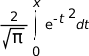# erf(), erff()

Compute the error function of a number

## Synopsis:

```#include <math.h>

double erf ( double x );

float erff ( float x );
```

## Arguments:

x
The number for which you want to compute the error function.

## Library:

libm

Use the -l m option to qcc to link against this library.

## Description:

The erf() and erff() functions compute the following:If x is large and the result of erf() is subtracted from 1.0, the results aren't very accurate; use erfc() instead.

This equality is true: erf(-x) = -erf(x)

## Returns:

The value of the error function, or NAN if x is NAN.

Note: If an error occurs, these functions return 0, but this is also a valid mathematical result. If you want to check for errors, set errno to 0, call the function, and then check errno again. These functions don't change errno if no errors occurred.

## Classification:

Safety:
Cancellation point No
Interrupt handler No
Signal handler No